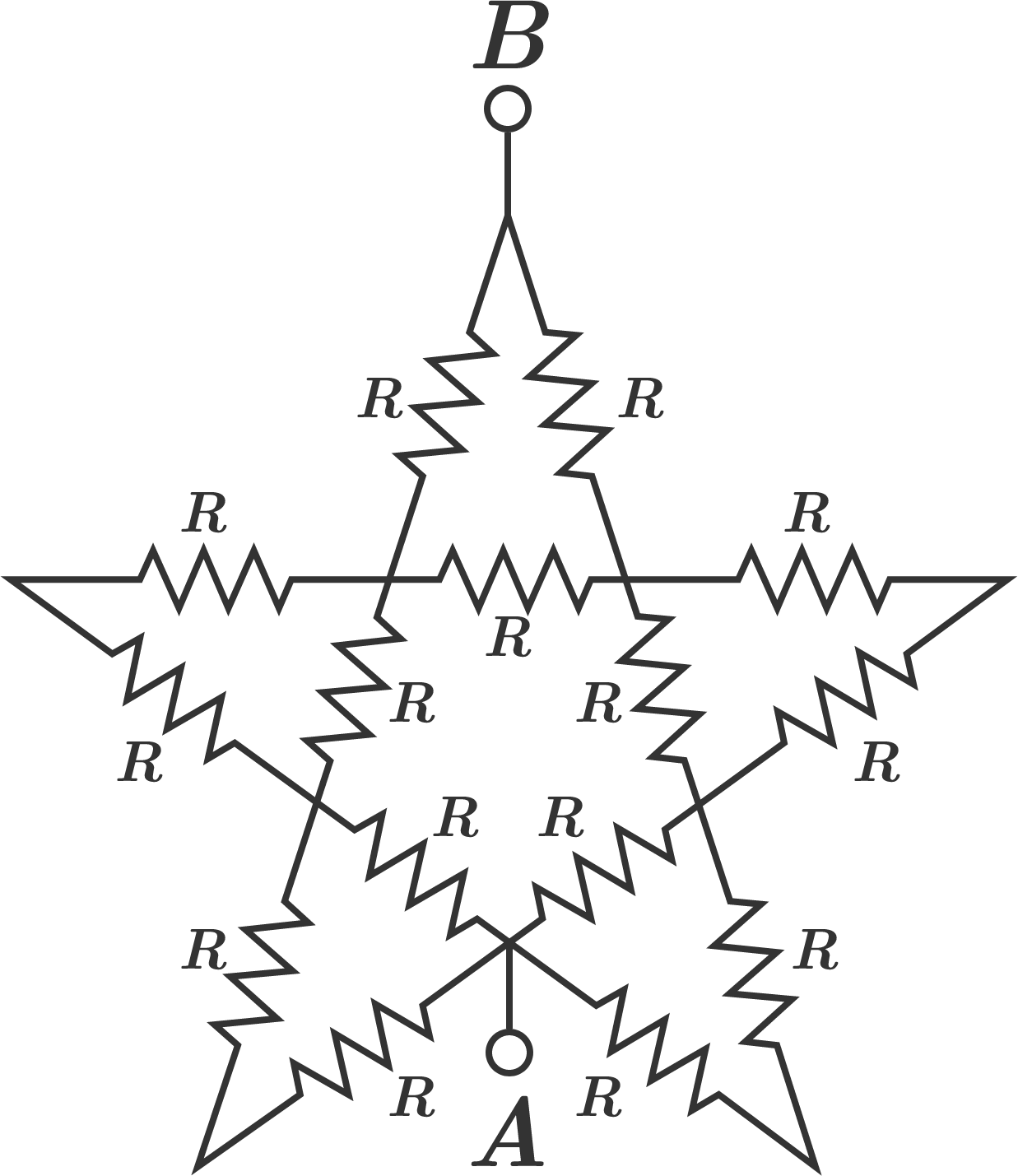# A Literal Star NetworkThe equivalent resistance between terminals $A$ and $B$ can be expressed as $\large{\frac{a}{b} R}$, where $a$ and $b$ are coprime positive integers.

Determine $a+b.$

×

Problem Loading...

Note Loading...

Set Loading...